# Intuitions on L1 and L2 Regularisation

This article was first published in Towards Data Science. Photo by rawpixel on Unsplash.

Overfitting is a phenomenon that occurs when a machine learning or statistics model is tailored to a particular dataset and is unable to generalise to other datasets. This usually happens in complex models, like deep neural networks.

Regularisation is a process of introducing additional information in order to prevent overfitting. The focus for this article is L1 and L2 regularisation.

There are many explanations out there but honestly, they are a little too abstract, and I’d probably forget them and end up visiting these pages, only to forget again. In this article, I will be sharing with you some intuitions why L1 and L2 work using gradient descent. Gradient descent is simply a method to find the ‘right’ coefficients through (iterative) updates using the value of the gradient. (This article shows how gradient descent can be used in a simple linear regression.)

Let’s go!

### 0) L1 and L2

L1 and L2 regularisation owes its name to L1 and L2 norm of a vector respectively. Here’s a primer on norms: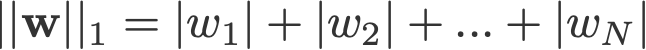1-norm (also known as L1 norm)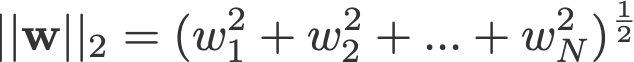2-norm (also known as L2 norm or Euclidean norm)p-norm

A linear regression model that implements L1 norm for regularisation is called lasso regression, and one that implements L2 norm for regularisation is called ridge regression. To implement these two, note that the linear regression model stays the same:

but it is the calculation of the loss function that includes these regularisation terms: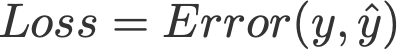Loss function with no regularisation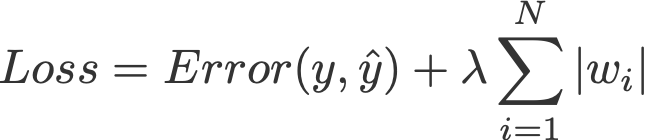Loss function with L1 regularisation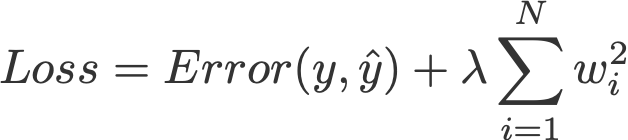Loss function with L2 regularisation

Note: Strictly speaking, the last equation (ridge regression) is a loss function with squared L2 norm of the weights (notice the absence of the square root). (Thank you Max Pechyonkin for highlighting this!)

The regularisation terms are ‘constraints’ by which an optimisation algorithm must ‘adhere to’ when minimising the loss function, apart from having to minimise the error between the true y and the predicted ŷ.

### 1) Model

Let’s define a model to see how L1 and L2 work. For simplicity, we define a simple linear regression model ŷ with one independent variable.

Here I have used the deep learning conventions w (‘weight’) and (‘bias’).

In practice, simple linear regression models are not prone to overfitting. As mentioned in the introduction, deep learning models are more susceptible to such problems due to their model complexity.

As such, do note that the expressions used in this article are easily extended to more complex models, not limited to linear regression.

### 2) Loss Functions

To demonstrate the effect of L1 and L2 regularisation, let’s define 3 loss functions:

• L
• L1
• L2

Our objective is to minimise these different losses.

#### 2.1) Loss function with no regularisation

We define the loss function L as the squared error, where error is the difference between y (the true value) and ŷ (the predicted value).

Let’s assume our model will be overfitted using this loss function.

#### 2.2) Loss function with L1 regularisation

Based on the above loss function, adding an L1 regularisation term to it looks like this:

where the regularisation parameter λ > 0 is manually tuned. Let’s call this loss function L1. Note that |w| is differentiable everywhere except when w=0, as shown below. We will need this later.

#### 2.3) Loss function with L2 regularisation

Similarly, adding an L2 regularisation term to L looks like this:

where again, λ > 0.

### 3) Gradient descent

Now, let’s solve the linear regression model using gradient descent optimisation based on the 3 loss functions defined aboveRecall that updating the parameter in gradient descent is as follows:

Let’s substitute the last term in the above equation with the gradient of LL1 and L2 w.r.t. w.

L:

L1:

L2:

### 4) How is overfitting prevented?

From here onwards, let’s perform the following substitutions on the equations above (for better readability):

• η = 1,
• H = 2x(wx+b-y)

which give us

L:

L1:

L2:

#### 4.1) With vs. Without Regularisation

Observe the differences between the weight updates with the regularisation parameter λ and without it.

Intuition A:

Let’s say with Equation 0, calculating w-H gives us a value that leads to overfitting. Then, intuitively, Equations {1.1, 1.2 and 2} will reduce the chances of overfitting because introducing λ makes us shift away from the very w that was going to cause us overfitting problems in the previous sentence.

Intuition B:

Let’s say an overfitted model means that we have a w value that is perfect for our model. ‘Perfect’ meaning if we substituted the data (x) back in the model, our prediction ŷ will be very, very close to the true y. Sure, it’s good, but we don’t want perfect. Why? Because this means our model is only meant for the dataset which we trained on. This means our model will produce predictions that are far off from the true value for other datasets. So we settle for less than perfect, with the hope that our model can also get close predictions with other data. To do this, we ‘taint’ this perfect w in Equation 0 with a penalty term λ. This gives us Equations {1.1, 1.2 and 2}.

Intuition C:

Notice that (as defined here) is dependent on the model (w and b) and the data (and y). Updating the weights based only on the model and data in Equation 0 can lead to overfitting, which leads to poor generalisation. On the other hand, in Equations {1.1, 1.2 and 2}, the final value of w is not only influenced by the model and data, but also by a predefined parameter λ which is independent of the model and data. Thus, we can prevent overfitting if we set an appropriate value of λ, though too large a value will cause the model to be severely underfitted.

#### 4.2) L1 vs. L2

We shall now focus our attention to L1 and L2, and rewrite Equations {1.1, 1.2 and 2} by rearranging their λ and H terms as follows:

L1:

L2:

Compare the second term of each of the equation above. Apart from H, the change in w depends on the ±λ term or the -2λw term, which highlight the influence of the following:

1. sign of current w (L1, L2)
2. magnitude of current w (L2)
3. doubling of the regularisation parameter (L2)

While weight updates using L1 are influenced by the first point, weight updates from L2 are influenced by all aspects. While I have made this comparison just based on the iterative equation update, please note that this does not mean that one is ‘better’ than the other.

For now, let’s see below how a regularisation effect from L1 can be attained just by the sign of the current w.

#### 4.3) L1’s effect on pushing towards 0 (sparsity)

Take a look at L1 in Equation 3.1. If w is positive, the regularisation parameter λ>0 will push w to be less positive, by subtracting λ from w. Conversely in Equation 3.2, if w is negative, λ will be added to w, pushing it to be less negative. Hence, this has the effect of pushing w towards 0.

This is of course pointless in a 1-variable linear regression model, but will prove its prowess to ‘remove’ useless variables in multivariate regression models. You can also think of L1 as reducing the number of features in the model altogether. Here is an arbitrary example of L1 trying to ‘push’ some variables in a multivariate linear regression model:

So how does pushing w towards 0 help in overfitting in L1 regularisation? As mentioned above, as goes to 0, we are reducing the number of features by reducing the variable importance. In the equation above, we see that x_2x_4 and x_5 are almost ‘useless’ because of their small coefficients, hence we can remove them from the equation. This in turn reduces the model complexity, making our model simpler. A simpler model can reduce the chances of overfitting.

#### Note

While L1 has the influence of pushing weights towards 0 and L2 does not, this does not imply that weights are not able to reach close to 0 due to L2.

### References

Norm (Mathematics) (wikipedia.org)

Lasso (Statistics) (wikipedia.org)

Lasso and Ridge Regularization (medium.com)

Special thanks to Yu Xuan, Ren Jie, Daniel and Derek for ideas, suggestions and corrections to this article. Also thank you C Gam for pointing out the mistake in the derivative.

Follow me on Twitter @remykarem or LinkedIn. You may also reach out to me via raimi.bkarim@gmail.com. Feel free to visit my website at remykarem.github.io.

## Author

•Raimi is an AI Engineer at AI Singapore. He likes building demos and write articles on machine learning.